# PSEB 6th Class Maths Solutions Chapter 4 Integers Ex 4.2

Punjab State Board PSEB 6th Class Maths Book Solutions Chapter 4 Integers Ex 4.2 Textbook Exercise Questions and Answers.

## PSEB Solutions for Class 6 Maths Chapter 4 Integers Ex 4.2

1. Using number line write the integer which is:

Question (a)
5 less than -1
Solution:
5 less than -1 = ?
We need to find the integer which is 5 less than -1.
So, we shall start with ‘-1 ’ and proceed 5 steps to the left of -1 as shown below: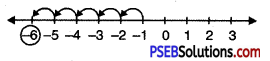Therefore 5 less than -1 is -6Question (b)
5 more than -5
Solution:
5 more than -5 = ?
We need to find the integer which is 5 more than -5.
So, we shall start with -5 and proceed 5 steps to the right of -5 as shown below: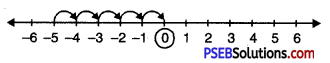Therefore 5 more than -5 is zero.

Question (c)
2 less than 5
Solution:
2 less than 5 = ?
We need to find the integer which is 2 less than 5.
2 steps to the left of 5 as shown below: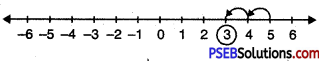Therefore 2 less than 5 is 3.

Question (d)
3 less than -2.
Solution:
3 less than – 2 = ?
We need to find the integer which is 3 less than -2.
So, we shall start with -2 and proceed 3 steps to the left of -2 as shown below: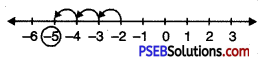Therefore 3 less than -2 is -5.2. Using number line, add the following integers:

Question (a)
9 + (-3)
Solution:
On number line we shall start from 0 and move 9 steps to the right of zero. Then we shall move three steps to the left of ‘+9’. We finally reach at +6. Thus, +6 is the answer.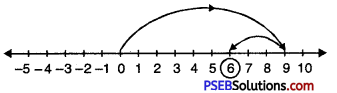Hence, 9 + (-3) = +6

Question (b)
5 + (-11)
Solution:
On number line we shall start from 0 and move 5 steps to the right of zero. Then we shall move eleven steps to the left of ‘+5’. We finally reach at ‘-6’. Thus, -6 is the answer.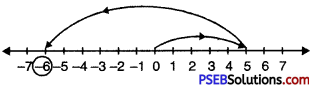Hence, 5 + (-11) = -6

Question (c)
(-1) + (-4)
Solution:
On number line we first move 1 step to the left of zero. Then we moved ahead 4 steps to the left of ‘-1\ We finally reaches at -5. Thus -5 is the answer.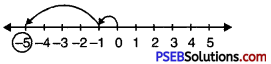Hence, (-1) + (-4) = -5

Question (d)
(-5) + 12
Solution:
On number line we shall move 5 steps to the left of zero. Then we shall move 12 steps to the right of ‘-5’ and finally reach at ‘+7’. Thus +7 is the answer.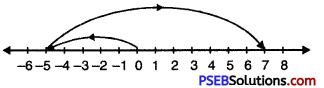Hence, (-5) + 12 = +7Question (e)
(-1) + (-2) + (-4)
Solution:
Step I: On number line we shall move one step to the left of zero suggested by minus sign of ‘-1’.
Step II: Then we shall move 2 steps to the left of -1 suggested by minus sign of -2 and we reach at ‘-3’.
Step III: Then again we shall move 4 steps to the left of ‘-3’ suggested by minus sign of -4 and finally we reach at-7.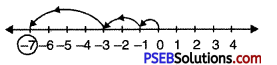Hence, (-1) + (-2) + (-4) = -7

Question (f)
(-2) + 4 + (-5)
Solution:
Step I: On number line we shall move 2 steps to the left of zero suggested by minus sign of ‘-2’.
Step II: Then we shall move 4 steps to the right of ‘-2’ suggested by plus sign of ‘44’ and we reach at +2.
Step III: Then we shall move 5 steps to the left of ‘+2’ as suggested by minus sign of ‘-5’ and finally we reach at -3. Thus answer is ‘-3’.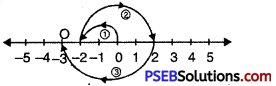Hence, (-2) + 4 + (-5) = -3

Question (g)
(-3) + (5) + (-4).
Solution:
Step I: On number line we shall move 3 steps to the left of zero as suggested by minus sign of ‘-3’.
Step II: Then we shall move 5 steps to the right of ‘-3’ as suggested by plus sign of ‘+5’ and we reach at ‘+2’.
Step III: Then we shall move 4 steps to the left of ‘+2’ as suggested by minus sign of ‘4’ and finally we reach at ‘-2’. Thus ‘-2’ is the answer.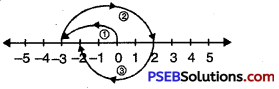Hence, (-3) + (5) + (-4) = -2

3. Add without using number line:

Question (a)
18 + 13
Solution:
18 + 13 = 31Question (b)
18 + (- 13)
Solution:
18 + (- 13) = + (18 – 13) = +5

Question (c)
(-18) + 13
Solution:
(-18) + 13 = – (18 – 13) = -5

Question (d)
(-18) + (-13)
Solution:
(-18) + (-13) = -(18 + 13) = -31

Question (e)
180 + (-200)
Solution:
180 + (-200) = -(200 – 180) = -20Question (f)
111 + (-67)
Solution:
111 + (-67) = (777 – 67) = 710

Question (g)
1262 + (-366) + (-962)
Solution:
1262 + (-366) + (-962)
= 1262 – (366 + 962)
= 1262 – 1328
= -(1328 – 1262) = -66

Question (h)
30 + (-27) + 21 + (-19) + (-3) + 11 + (-9)
Solution:
30 + (-27) + 21 + (-19) + (-3) + 11 + (-9)
= 30 + 21 + 11 + (-27) + (-19) + (-3) + (-9)
= 62 + (-58) = 62 – 58 = 4

Question (i)
(-7) + (-9) + 4 + 16
Solution:
(-7) + (-9) + 4+16 = (-16) + 20 = 4Question (j)
37 + (-2) + (-65) + (-8).
Solution:
37 + (-2) + (-65) + (-8)
= 37 – (2 + 65 + 8)
= 37 – 75 = -38

4. Write the successor and predecessor of the following:

Question (a)
-15
Solution:
Successor of -15 = -15 + 1 = -14
Predecessor of -15 = -15 – 1 = -16

Question (b)
27
Solution:
Successor of 27 = 27 + 1 = 28
Predecessor of 27 = 27 – 1 = 26

Question (c)
-79
Solution:
Successor of -79 = -79 + 1 = -78
Predecessor of -79 = -79- 1 = -80Question (d)
0
Solution:
Successor of 0 = 0+ 1 = 1
Predecessor of 0 = 0 – 1 = -1

Question (e)
29
Solution:
Successor of 29 = 29 + 1 = 30
Predecessor of 29 = 29 – 1 = 28

Question (f)
-18
Solution:
Successor of -18 = -18 + 1 = -17
Predecessor of -18 = -18 – 1 = -19

Question (g)
-21
Solution:
Successor of -21 = -21 + 1 = -200
Predecessor of -21 = -21 – 1 = -22

Question (h)
99
Solution:
Successor of 99 = 99 + 1 = 100
Predecessor of 99 = 99 – 1 = 98

Question (i)
-1
Solution:
Successor of-1 = -1 + 1 = 0
Predecessor of -1 = -1 – 1 = -2

Question (j)
-13.
Solution:
Successor of -13 = -13 + 1 = -12
Predecessor of -13 = -13 – 1 = -145. Complete the following addition table:

 + -3 -4 -2 +1 +2 +3 -2 -3 0 +1 +2

Solution:

 + -3 -4 -2 + 1 +2 +3 -2 -5 -6 -4 -1 0 + 1 -3 -6 -7 -5 -2 -1 0 0 -3 -4 -2 + 1 +2 +3 + 1 -2 -3 -1 +2 +3 +4 +2 -1 -2 0 +3 +4 +5